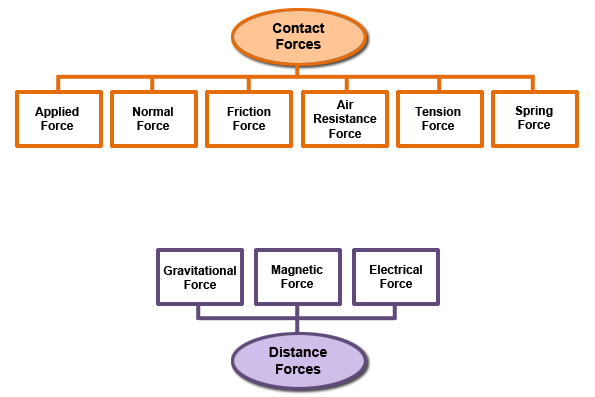An applied force is a force that is applied to an object by a person or another object. An example is a person pushing a desk across the room. The person is exerting the force on the desk.

Normal force is the support force applied to an object that is in contact with another stable object. An example would be a book resting on a table. The table is exerting an upward force on the book.

Friction force is the force that one object exerts in another when the two rub against each other. Most of the time, friction force opposes the motion of an object. For example, if you roll a ball across the carpet, there is friction between the ball and the carpet; this slows the ball down.

The air resistance is a special type of frictional force that acts upon objects as they travel through the air. For example, when a skydiver falls, there is air resistance pushing on him or her.

The tension force is the force that is transmitted through a string, rope, cable or wire when it is pulled tight by forces acting from opposite ends. Tug of war is an example of tension force.

The spring force is the force exerted by a compressed or stretched spring upon any object that is attached to it. Windup toys are able to move because of spring force. Inside the toy is a spring. When it is wound and released, the force causes the toy to move.

The force of gravity is the force with which the earth, moon, or other massively large object attracts another object towards itself. Gravitational force is responsible for holding the planets in orbit around the sun.

Magnetic force is the attraction between two magnetic objects. An example is placing a magnet on a refrigerator.

Electrical force is the force exerted due to the motion of electrons. For example, when you walk across carpet in your socks and you touch another object, you will get a small shock. That shock is a result of electrical force.

Contact forces are forces that result when two interacting objects are physically contacting (touching) each other.

Distance forces are forces that result when the two interacting objects are not in physical contact with each other, yet are able to exert a push or pull despite their physical separation.